June 27, 2022, 03:25:40 pm
 News: Welcome to Legendary Squad's Forums!Home Help Search Gallery Chat Staff List Login Register

# [Coding] ynvaser's Helper Projectile Tutorial

 Pages:    Go DownAuthor Topic: [Coding] ynvaser's Helper Projectile Tutorial  (Read 3662 times)
ynvaser
I'm awesome
Ultimate Sayan Of Legends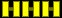Honor: +1/-0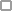Offline

Gender: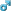Posts: 1010I have an offer. GIVE FEEDBACK OR DIE!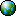« on: October 30, 2008, 04:30:04 am »

Hi guys!

This is my very first tutorial. It explains a kind of easy thing, a helper used as a projectile.
It has many benefits, since you can create very crazy things, like the projectile follows the enemy, or blows up and creates many frags, etc. In this tutorial, you will learn the simplest (plain projectile with random direction) To understand this, you will need to know the basics, such as summoning helpers, making anims, creating a simple move, and knowing what's what. If you don't know these, either read  Cyanide's Narutorials, or try to figure the basics out from Elecbyte's tutorials, located in your docs folder of MUGEN.

You must add this to your character's projectile launcher state (where it shots):
;...
[State 420, Helper]         ;This summons helper
type = Helper
trigger1 = Time = 11        ;It summons when the state is at the 11th tick. Change as needed.
helpertype = normal         ;Normal, so not player XD
name = "Renzoku Projectile" ;Give it a name, it's a good thing.
ID = 9001                   ;The indentification number of the helper
stateno = 9001              ;The helper's stateno. If you change it in the helper's state, change it here too.
pos = 50, -44               ;The position where the helper appears
postype = p1                ;It defines from where it counts the distance.
facing = 1                  ;1 is foward, -1 is backward. We want it foward.
keyctrl = 0                 ;Keep this at 0.
ownpal = 1                  ;This means that the parent's PalFX's won't affect the helper.
;...

Here's my Goku's Renzoku Projectile State (Copy/Pasteable):
;-Renzoku Projectile   ;I like to give them names
;(Used in StateNo 420) ;This is good to keep your helpers organized
[Statedef 9001]        ;Change the value to fit your needs
type = A
movetype = A
physics = N
anim = 9014            ;Change the anim to your projectile's anim. It must contain at least 1 clsn1 box.
velset = 0,0
ctrl = 0
juggle = 10            ;Change this to fit your char
facep2 = 0
hitdefpersist = 0
movehitpersist = 0
hitcountpersist = 0
sprpriority = 3       ;Adjust it, if you want the proj to be behind or in front of everyone.

[State 9001, AssertSpecial]  ;This sctrl won't let the helper's shadow cast.
type = AssertSpecial
trigger1 = 1

[State 9001, PlayerPush] ;This disables the clsn2's pushing effect.
type = PlayerPush
trigger1 = 1
value = 0

[State 9001, ScreenBound];I totally forgot to include this code, sorry for that
type = ScreenBound;This will prevent the projectiles to collide with thew edge of the level
trigger1 = 1
value = 0
movecamera = 0,0

[State 9005, Hitoverride];This will change your projectile into a specific state when getting hit
type = HitOverride
trigger1 = 1
attr = SCA, AA,AP,AT
time = -1
stateno = 9000;The number of your projectile's hitstate

;Don't change the order of the following 3 sctrl's.
[State 9001, VarRandom] ;This var randomizes the y velocity of the helper.
type = VarRandom
trigger1 = (!Time)
v = 0
range = -2,2

[State 9001, VarRandom] ;This var serves as a speed booster to the x velocity.
type = VarRandom
trigger1 = (!Time)
v = 1
range = 2,0

[State 9001, VelSet] ;This sctrl sets the velocity of the helper.
type = VelSet
trigger1 = (!Time)
x = 15+(Var(1))      ;As you see, the base velocity is 15, but with the +var(1), it's either 15,16, or 17.
y = (Var(0))         ;There's no base, the var(0) defines the y velocity completely.

[State 9001, HitDef] ;The heart of The code. It defines how the the projectile hits.*
type = HitDef        ;*Because i suppose you know the basics, I won't bother explain all.
trigger1 = 1
attr = S, NA
hitflag   = MAFDP
guardflag = MA
animtype = Hard
priority = 7, Hit
damage = 10,5
pausetime = 5,5
guard.pausetime   = 15,20
sparkno   = S6150
guard.sparkno = S6150
sparkxy   = 0,40
hitsound = S1,12
guardsound = S1,12
ground.type = High
air.type = Trip
ground.slidetime = 25
ground.hittime = 25
air.hittime   = 25
ground.velocity   = -8
guard.velocity = -8
air.velocity = -8,-3.5
airguard.velocity = -8,-3.5
ground.cornerpush.veloff = 0
fall.xvelocity = -1
fall.yvelocity = -3
fall.recover = 0
air.fall = 1
down.velocity = -1,-2
down.bounce   = 1
id = 9001
getpower = 0
givepower = 25
palfx.time = 12
palfx.mul = 256,256,256
envshake.time = 12
envshake.freq = 120
envshake.ampl = -4
persistent = 0

[State 9001, DestroySelf]              ;The finale. It destroys the helper in specific circumstances
type = DestroySelf
trigger1 = MoveContact             ;First condition. It destroys when the projectile hits.
trigger2 = FrontEdgeBodyDist < -30 ;Second condition. It destroys when the x distance from the front*
ignorehitpause = 1                       ;*vertical wall is smaller than -30 (so when the distance is -31 or smaller)

;Helper Destroy State;You can notice that this is a new state, the name explains why it stands for
;(Used in Stateno 9001)
[Statedef 9000]
type       = S
movetype   = I
physics      = N
anim      = -1
velset      = 0,0
ctrl      = 0
juggle      = 0
facep2      = 0
hitdefpersist   = 0
movehitpersist   = 0
hitcountpersist   = 0
sprpriority   = 0

[State 9000, DestroySelf];Destroys the helper instantly
type = DestroySelf
trigger1 = Time = 0
ignorehitpause = 1

If you used this tutorial in your character, I would like to see my name in the credits list, and if you want, you could mail me so I can keep track of where this is used.
I hope you learned much from it!
 Report SpamLogged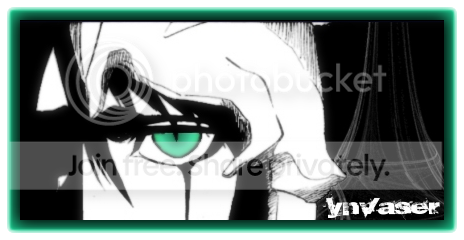ynvaser's effect pack / Goku / Broly || My W.I.P.s

 Pages:    Go Up